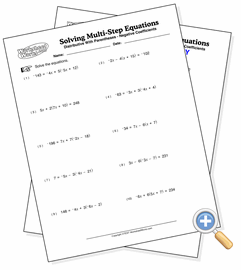# Using Distributive Property

## Solving Multi-Step EquationsSolve equations involving the distributive property of multiplication

These equations require the student to distribute a multiplied value across terms within parentheses before combining like terms. It is usually not easy to do it in any other way. All problems resolve to integers. You can adjust the difficulty of the problems by increasing the values size, using negative coefficients and flipping the sides of the equations.